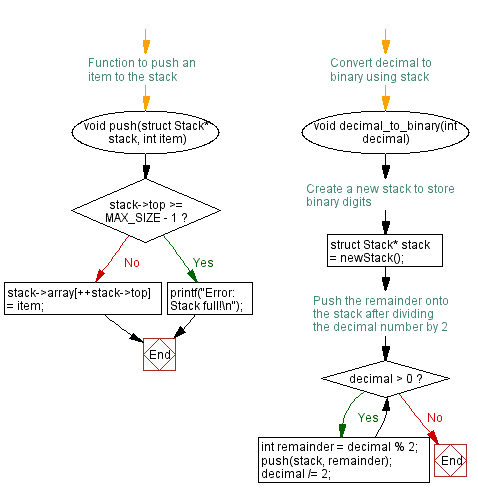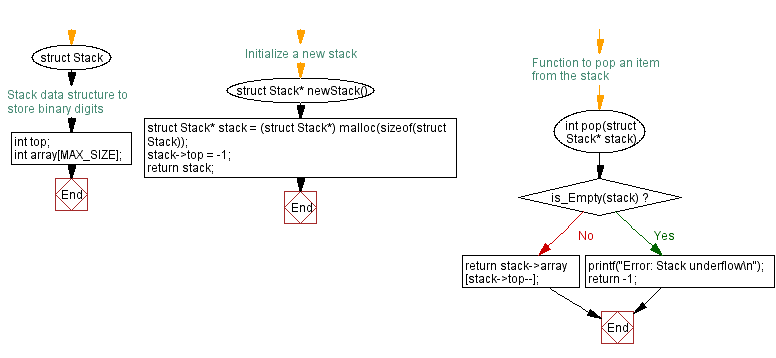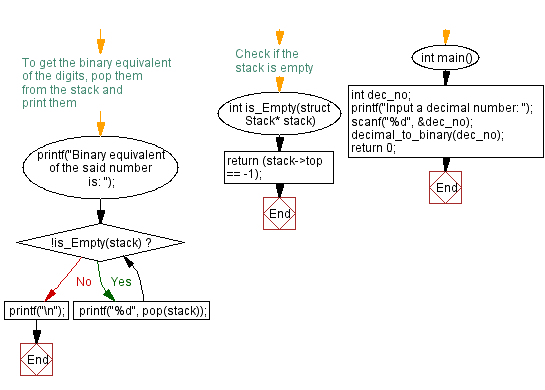﻿ C - Decimal number to its binary equivalent using stack

# C Exercises: Decimal number to its binary equivalent using stack

## C Stack: Exercise-17 with Solution

Write a C program to convert a decimal number to its binary equivalent using stack.

Sample Solution:

C Code:

``````#include <stdio.h>
#include <stdlib.h>

#define MAX_SIZE 100

// Stack data structure to store binary digits
struct Stack {
int top;
int array[MAX_SIZE];
};
// Initialize a new stack
struct Stack* newStack() {
struct Stack* stack = (struct Stack*) malloc(sizeof(struct Stack));
stack->top = -1;
return stack;
}
// Check if the stack is empty
int is_Empty(struct Stack* stack) {
return (stack->top == -1);
}

// Function to push an item to the stack
void push(struct Stack* stack, int item) {
if (stack->top >= MAX_SIZE - 1) {
printf("Error: Stack full!\n");
return;
}
stack->array[++stack->top] = item;
}
// Function to pop an item from the stack
int pop(struct Stack* stack) {
if (is_Empty(stack)) {
printf("Error: Stack underflow\n");
return -1;
}
return stack->array[stack->top--];
}
// Convert decimal to binary using stack
void decimal_to_binary(int decimal) {
// Create a new stack to store binary digits
struct Stack* stack = newStack();
// Push the remainder onto the stack after dividing the decimal number by 2
while (decimal > 0) {
int remainder = decimal % 2;
push(stack, remainder);
decimal /= 2;
}
// To get the binary equivalent of the digits, pop them from the stack and print them
printf("Binary equivalent of the said number is: ");
while (!is_Empty(stack)) {
printf("%d", pop(stack));
}
printf("\n");
}
int main() {
int dec_no;
printf("Input a decimal number: ");
scanf("%d", &dec_no);
decimal_to_binary(dec_no);
return 0;
}
```
```

Sample Output:

```Input a decimal number: 10
The binary equivalent is: 1010
Input a decimal number: 109
Binary equivalent of the said number is: 1101101

Input a decimal number: 2015
Binary equivalent of the said number is: 11111011111
```

Flowchart:C Programming Code Editor:

Have another way to solve this solution? Contribute your code (and comments) through Disqus.

What is the difficulty level of this exercise?

Test your Programming skills with w3resource's quiz.

﻿

## C Programming: Tips of the Day

C Programming - How do you pass a function as a parameter in C?

Declaration

A prototype for a function which takes a function parameter looks like the following:

```void func ( void (*f)(int) );
```

This states that the parameter f will be a pointer to a function which has a void return type and which takes a single int parameter. The following function (print) is an example of a function which could be passed to func as a parameter because it is the proper type:

```void print ( int x ) {
printf("%d\n", x);
}
```

Function Call

When calling a function with a function parameter, the value passed must be a pointer to a function. Use the function's name (without parentheses) for this:

```func(print);
```

would call func, passing the print function to it.

Function Body

As with any parameter, func can now use the parameter's name in the function body to access the value of the parameter. Let's say that func will apply the function it is passed to the numbers 0-4. Consider, first, what the loop would look like to call print directly:

```for ( int ctr = 0 ; ctr < 5 ; ctr++ ) {
print(ctr);
}
```

Since func's parameter declaration says that f is the name for a pointer to the desired function, we recall first that if f is a pointer then *f is the thing that f points to (i.e. the function print in this case). As a result, just replace every occurrence of print in the loop above with *f:

```void func ( void (*f)(int) ) {
for ( int ctr = 0 ; ctr < 5 ; ctr++ ) {
(*f)(ctr);
}
}
```

Ref : https://bit.ly/3skw9Um